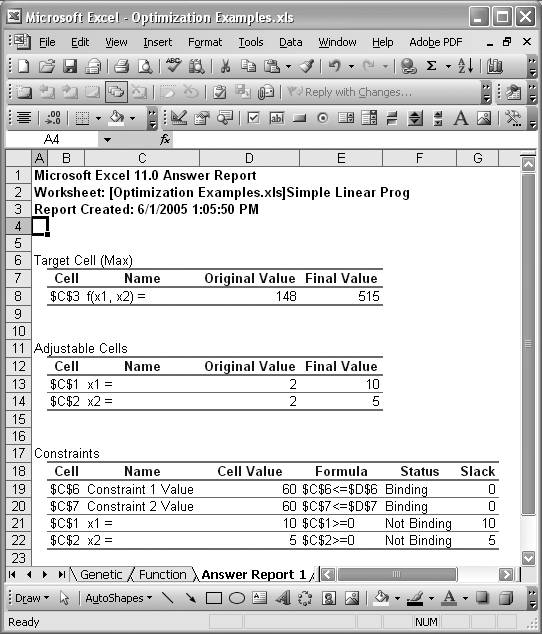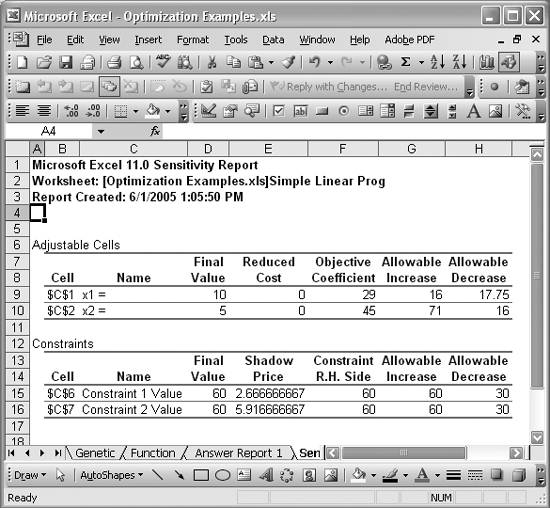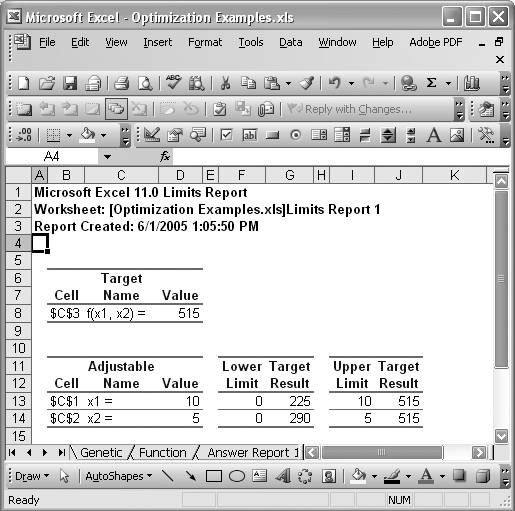# Understanding Solver Reports

Problem

You know that Solver can generate several types of reports upon finding a solution to a problem and you'd like to learn more about these.

Solution

The version of Solver that ships with Excel can generate three reports: an Answer report, a Sensitivity report, and a Limits report. These reports are described in the following discussion.

Discussion

Solver's three reports are helpful when interpreting the results obtained by Solver. When it finds a solution, you're presented with a dialog box containing a message regarding the nature of the solution found. You can choose which Solver reports , if any, to generate. (See the introduction to Chapter 9 for more information.) Solver will generate the selected reports in separate worksheets that it will create automatically. Each of the three available report types is explained in the following sections.

Figure 13-15 shows an example Answer report. This is the Answer report for the problem solved in Recipe 13.1.

Answer reports show the initial and final values of the objective (Target Cell) and all variables (Adjustable Cells). You can quickly see from these reports how close your initial guess was to the optimal solution.

This report also shows the optimum values for all constraints, including a note indicating which constraints were actually binding. For Not Binding constraints, the amount of slack is also reported. The slack on a constraint tells you how far away a constraint is from becoming a binding constraint. All this information is helpful in determining which constraints govern, or limit, the problem being solved, and how much leeway you have on other constraints.

Notice that these reports contain cell references to the objective, variables, and constraints. They also include a name for these cells. These are not cell names as defined using Excel's cell name feature (discussed in Recipe 1.14). Instead, these names are automatically generated by Solver using a specific algorithm. If you set your spreadsheet up with this algorithm in mind, the names are meaningful; otherwise, they could be completely meaningless.Solver builds names for target, variable, and constraint cells by searching to the left of the given cell for the first cell containing text. At the same time, it looks above the given cell for the first cell containing text. The text in these two cells is combined to create the name shown in Solver reports. Therefore, if you want a cell to have a meaningful label, whether it's a cell containing the objective function, a variable, or a constraint, you need to make sure that the text cells nearest to the left of and above the given cell contain the desired label. I typically put text labels to the left of my target, variable, or constraints cells, and make sure no cell above these has any text.

Sensitivity report

There are two versions of the sensitivity report. The one shown in Figure 13-16 corresponds to a linear problem. Solver generates a different sensitivity report for nonlinear problems, as shown in Figure 13-17. These reports tell you how sensitive the solution is to small changes in variables and constraints.

Figure 13-16. Linear sensitivity reportReferring to Figure 13-16, you will see that the linear problem sensitivity report contains two tables. The first table provides information on the sensitivity of the problem's variables, while the second contains information on the sensitivity of the problem's constraints.

Both tables start with a cell reference column, along with a label and then a final value column. The final values are simply the resulting values of the variables or constraints corresponding to the solution found by Solver. The other columns in these tables give you the sensitivity information.

The Reduced Cost applies to variables whose optimum value turns out to be 0. In this case, reduced cost provides an estimate of how much the objective function will change if you force the variable to assume some nonzero value. Variables whose values turn out to be nonzero for the optimum solution will show a reduced cost of 0.

Figure 13-17. Nonlinear sensitivity reportThe Objective Coefficient shows the coefficient corresponding to each variable as it appears in the objective function. The Allowable Increase and Allowable Decrease values tell you that so long as these coefficients remain with their current value plus or minus the allowable increase and decrease, respectively, the optimum values for the variables will remain unchanged, although the optimum value for the objective function (target cell) may change.

The Shadow Price for each constraint shown in the Constraints table tells you how much the value of the objective function will change if the value of the righthand side of the constraint, shown in the Constraint R.H. Side column, changes within the limits specified by the Allowable Increase and Allowable Decrease values.

For nonlinear problems, the sensitivity report is a little different. Figure 13-17 shows the sensitivity report for a nonlinear problem.

As in the linear case, there are two tables, corresponding to variables (Adjustable Cells) and constraints. In both cases the cell reference, name, and final optimum values for each variable and constraint are shown.

For variables, the Reduced Gradient tells you how much the value of the objective function would change if the value of the variable were increased by 1. For constraints, the Lagrange Multiplier tells you how much the value of the objective function would change if the righthand-side value of the constraint were increased by 1.

Limits report

Limits reports tell you how the value of the objective function changes as each variable is maximized and minimized, while all other values are held constant and while still satisfying the problem's constraints. Figure 13-18 shows a typical Limits report.

Figure 13-18. Limits reportThe Target values show you the value of the objective function corresponding to the lower and upper limits of the variables obtained through a maximization and minimization process as described a moment ago.Excel Scientific and Engineering Cookbook (Cookbooks (OReilly))
ISBN: 0596008791
EAN: 2147483647
Year: N/A
Pages: 206
Authors: David M Bourg